# Slope of a Line

In geometry, we have seen the lines drawn on the coordinate plane. To predict whether the lines are parallel or perpendicular or at any angle without using any geometrical tool, the best way to find this is by measuring the slope. In this article, we are going to discuss what a slope is, slope formula for parallel lines, perpendicular lines, slope for collinearity with many solved examples in detail.

## What is a Slope?

In Mathematics, a slope of a line is the change in y coordinate with respect to the change in x coordinate.

The net change in y-coordinate is represented by Δy and the net change in x-coordinate is represented by Δx.

Hence, the change in y-coordinate with respect to the change in x-coordinate is given by,

m = change in y/change in x = Δy/Δx

Where “m” is the slope of a line.

The slope of the line can also be represented by

tan θ = Δy/Δx

So, tan θ to be the slope of a line.

Generally, the slope of a line gives the measure of its steepness and direction. The slope of a straight line between two points says (x1,y1) and (x2,y2) can be easily determined by finding the difference between the coordinates of the points. The slope is usually represented by the letter ‘m’.

## Slope Formula

If P(x1,y1) and Q(x2,y2) are the two points on a straight line, then the slope formula is given by:

 Slope, m = Change in y-coordinates/Change in x-coordinates m = (y2 – y1)/(x2 – x1)

Therefore, based on the above formula, we can easily calculate the slope of a line between two points.

In other term, the slope of a line between two points is also said to be the rise of the line from one point to another (along y-axis) over the run (along x-axis). Therefore,

Slope, m = Rise/Run

## Slope of a Line Equation

The equation for the slope of a line and the points also called point slope form of equation of a straight line is given by:

 y − y1 = m(x − x1)

Whereas the slope-intercept form the equation of the line is given by:

y = mx + b

Where b is the y-intercept.

## How to Find Slope of a Line on a Graph?

In the given figure, if the angle of inclination of the given line with the x-axis is θ then, the slope of the line is given by tan θ. Hence, there is a relation between the lines and angles. In this article, you will learn various formulas related to the angles and lines.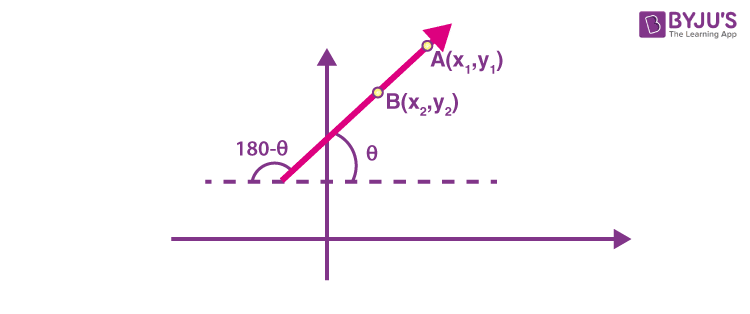The slope of a line is given as m = tan θ. If two points A (x1, y1) and B(x2, y2) lie on the line with x1 ≠ x2 then the slope of the line AB is given as:

$$\begin{array}{l} m = tan\ \theta =\frac{y_2~-~y_1}{x_2~-~x_1}\end{array}$$

Where θ is the angle which the line AB makes with the positive direction of the x-axis. θ lies between 0° and 180°.

It must be noted that θ = 90° is only possible when the line is parallel to y-axis i.e. at x1 = x2  at this particular angle the slope of the line is undefined.

Conditions for perpendicularity, parallelism, and collinearity of straight lines are given below:

## Slope for Parallel Lines

Consider two parallel lines given by l1 and l2 with inclinations α and β respectively. For two lines to be parallel their inclination must also be equal i.e. α=β. This results in the fact that tan α = tan β. Hence, the condition for two lines with inclinations α, β to be parallel is tan α = tan β.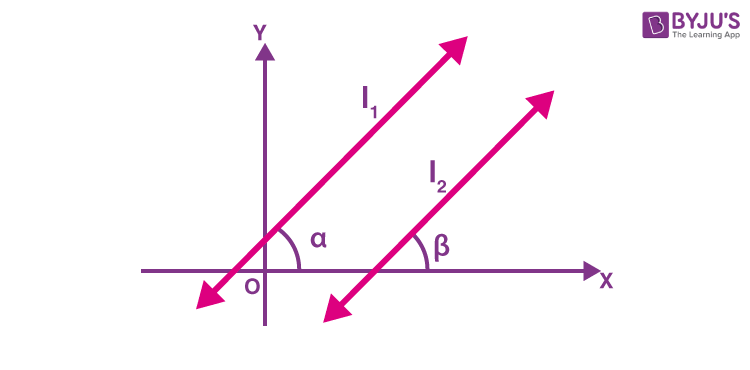Therefore, if the slopes of two lines on the Cartesian plane are equal, then the lines are parallel to each other.

Thus, if two lines are parallel then, m1 = m2.

Generalizing this for n lines, they are parallel only when the slopes of all the lines are equal.

If the equation of the two lines are given as ax + by + c = 0 and a’ x + b’ y + c’= 0, then they are parallel when ab’ = a’b. (How? You can arrive at this result if you find the slopes of each line and equate them.)

## Slope for Perpendicular Lines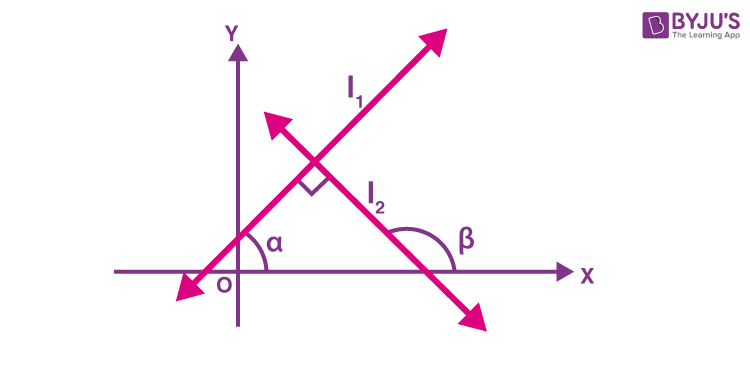In the figure, we have two lines l1 and l2 with inclinations α, β. If they are perpendicular, we can say that β = α + 90°. (Using properties of angles)

Their slopes can be given as:

m1 = tan(α + 90°) and m2 = tan α.

$$\begin{array}{l}\Rightarrow m_1 = – cot\ \alpha = -~ \frac{1}{tan~\alpha} = -~\frac {1}{m_2}\end{array}$$
$$\begin{array}{l}\Rightarrow m_1 = -\frac {1}{m_2} \end{array}$$
$$\begin{array}{l}\Rightarrow m_1 ~\times ~m_2 = -1\end{array}$$

Thus, for two lines to be perpendicular the product of their slope must be equal to -1.

If the equations of the two lines are given by ax + by + c = 0 and a’ x + b’ y + c’ = 0, then they are perpendicular if, aa’+ bb’ = 0. (Again, you can arrive at this result if you find the slopes of each line and equate their product to -1.)

## Slope for Collinearity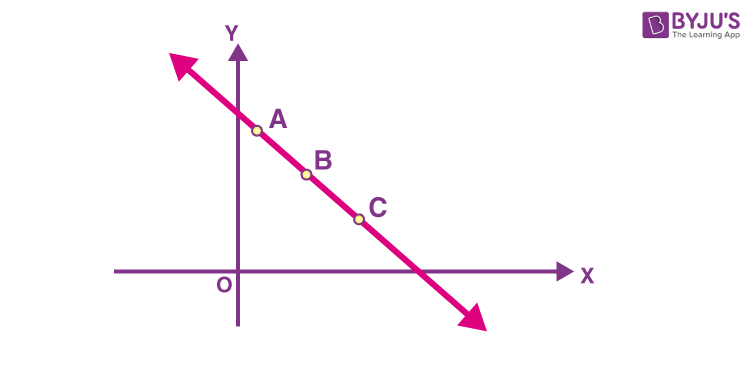For two lines AB and BC to be collinear the slope of both the lines must be equal and there should be at least one common point through which they should pass. Thus, for three points A, B, and C to be collinear the slopes of AB and BC must be equal.

If the equation of the two lines is given by ax + by + c = 0 and a’ x+b’ y+c’ = 0, then they are collinear when ab’ c’ = a’ b’ c = a’c’b.

## Angle between Two Lines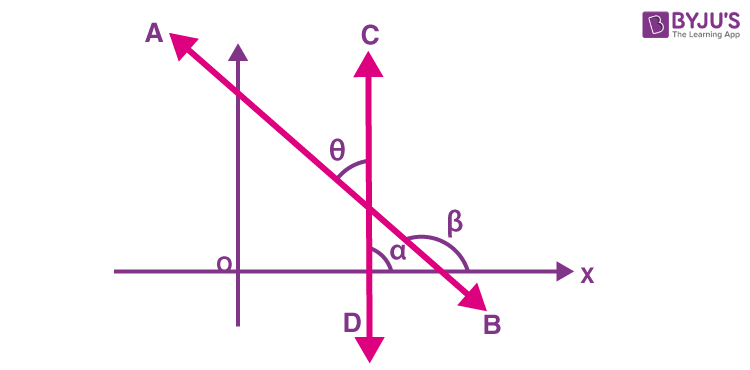When two lines intersect at a point then the angle between them can be expressed in terms of their slopes and is given by the following formula:

$$\begin{array}{l}tan\ \theta = | \frac{ m_2~-~m_1}{1~+~m_1~ m_2}|\end{array}$$

where m1 and m2 are the slopes of the line AB and CD respectively.

$$\begin{array}{l}\text{If } \frac{ m_2~-~m_1}{1~+~m_1~ m_2} \text{ is positive then the angle between the lines is acute.}\end{array}$$
$$\begin{array}{l}\text{If } \frac{ m_2~-~m_1}{1~+~m_1~ m_2} \text{ is negative then the angle between the lines is obtuse.}\end{array}$$

## Slope of Vertical Lines

Vertical lines have no slope, as they do not have any steepness. Or it can be said, we cannot define the steepness of vertical lines.

A vertical line will have no values for x-coordinates. So, as per the formula of slope of the line,

Slope, m = (y2 – y1)/(x2 – x1

But for vertical lines, x2 = x1 = 0

Therefore,

m = (y2 – y1)/0 = undefined

In the same way, the slope of horizontal line is equal to 0, since the y-coordinates are zero.

m = 0/(x2 – x1) = 0 [for horizontal line]

## Positive and Negative Slope

If the value of slope of a line is positive, it shows that line goes up as we move along or the rise over run is positive.

If the value of slope is negative, then the line goes done in the graph as we move along the x-axis.

### Solved Examples on Slope of a Line

Example 1:

Find the slope of a line between the points P = (0, –1) and Q = (4,1).

Solution:

Given,the points P = (0, –1) and Q = (4,1).

As per the slope formula we know that,

Slope of a line, m = (y2 – y1)/(x2 – x1

m = (1-(-1))/(4-0) = 2/4 = ½

Example 2:

Find the slope of a line between P(–2, 3) and Q(0, –1).

Solution:

Given,  P(–2, 3) and Q(0, –1) are the two points.

Therefore, slope of the line,

m = (-1-3)/0-(-2) = -4/2 = -2

Example 3:

Ramya was checking the graph, and she realized that the raise was 10 units and the run was 5 units. What should be the the slope of a line?

Solution:

Given that, Raise = 10 units

Run = 5 units.

We know that the slope of a line is defined as the ratio of raise to the run.

i.e. Slope, m = Raise/Run

Hence, slope = 10/5 = 2 units.

Therefore, the slope of a line is 2 units.

Stay tuned with BYJU’S – The Learning App and download the app to learn all Maths-related concepts easily by exploring more videos.

## Frequently Asked Questions on Slope of a Line

Q1

### What is the slope of a straight line?

The slope expresses the steepness and direction of the line. It represents how steep a line is.
Q2

### What are the three different ways to find the slope?

The slope can be found using different methods, such as standard form, slope-intercept form, and point-intercept form.
Q3

### What is the point-slope equation of a straight line?

The point-slope form of the equation of a straight line is given by:
y − y1 = m(x − x1)
Q4

### How to find slope of a line?

We need to find the ratio of the difference between the y-coordinates and x-coordinates of the two points, that form the line. The resulted value is the slope of the line. It shows the rise of the line along the y-axis over the run along x-axis.
Q5

### What is the slope between two points?

The slope between two points is calculated by evaluating the change in y-coordinate values and x-coordinate values. For example, the slope between the points (4,8) and (-7,1) is equal to:
m = (1-8)/(-7-4) = -7/-11 = 7/11
Q6

### What is the slope of the line: y = −2x + 7?

The slope of the line whose equation is y = -2x + 7 is -2.
m = -2
Quiz on Slope of a Line